One of the specialty areas of the RCMP is accident reconstruction.  These people analyze traffic accidents to determine the cause of an accident and the causes of any injuries that people sustained.  It is also their job to decide whether or not the accident was preventable.  To do this, they need to have a very acute sense of mathematics.

Frequently, when a collision accident happens, there are skid marks left on the roadway from a vehicle that has locked its breaks.  Specialists measure the skid marks and apply the measurement to a formula that enables them to find the speed of the vehicle, prior to the collision.  The formula that the accident re-constructionists use is: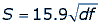S is the speed of the vehicle, d is the distance of the skid marks, and f is the coefficient of friction.  Measuring the distance of skid marks is a fairly routine procedure and can be done relatively easily, but it is a little more difficult to find the coefficient of friction.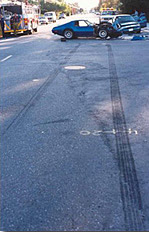Image reproduced with permission of Surrey RCMP

The coefficient of friction is the amount of friction (force) acting on the tires, and is influenced by the weight of the vehicle, the roadway surface (asphalt, gravel, etc.) and weather conditions (rain or ice).   The coefficient of friction is measured as a force and can be found using the formula: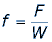The f is the measurement of force (the coefficient of friction), the F is the force of gravity coming down on the tires, and W is the weight of the tire.  In order to find the force of gravity (sometimes referred to as the drag factor), specialists actually drag one of the weighed tires along the roadway to determine the force.  They will drag the tire maybe twenty times and take the average force measurement.  To actually find the measurement of the drag factor requires a number of complex formulas and some special equipment.
In order to use this formula for measuring skid marks, the skids have to be in straight lines.

Sometimes, vehicles will skid in a circular motion.  These marks are called yaw marks.  Yaw marks occur when tires rotate and spin.  In order to find the speed the vehicle was traveling before it started to skid, accident re-constructionists use another formula: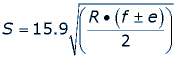S is the speed of the vehicle before impact, f is the coefficient of friction, e is the elevation of the road, and R is the radius of the yaw mark.  The radius is determined by finding the centre of mass of one of the tires, which acts as the centre of the circle.  This formula, one of many more complex formulas involved in this problem, utilizes the understanding of linear momentum as the vehicle spins out of control.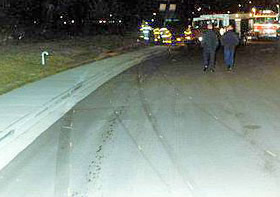Image reproduced with permission of Surrey RCMP

Because of the Law of Conservation of Momentum, energy is neither created nor destroyed. Thus, if specialists know the angles that the incoming vehicles collided at and the weight of the vehicles, they can determine the speeds of the vehicles.

Equations are also used to find speeds of vehicles using other information, aside from skid and yaw marks.  If a pedestrian is hit, or a vehicle rolls, speeds can be determined by analyzing the movement of the pedestrian and vehicle.  If a vehicle or pedestrian vaults, flips, or falls, these differences can help determine the speed of the traveling vehicle.  Again, specialists use a number of complicated equations to find an exact speed.  After an accident happens, officials can use math to decide who was driving the vehicle, even if the driver was not wearing a seatbelt, by looking at where the victims landed and then measuring and figuring out angles.  There is only one solution.